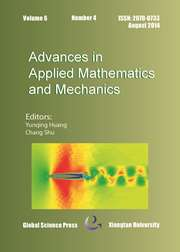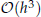Home
Hostname: page-component-66d7dfc8f5-tfp9r Total loading time: 0.242 Render date: 2023-02-08T21:57:25.976Z Has data issue: true Feature Flags: { "useRatesEcommerce": false } hasContentIssue trueAdvances in Applied Mathematics and Mechanics

# Optimal Bicubic Finite Volume Methods on Quadrilateral Meshes

Published online by Cambridge University Press:  29 May 2015

*
*Corresponding author. Email: chenyanli7532@sina.com (Y. L. Chen), yonghai@jlu.edu.cn (Y. H. Li)

## Abstract

In this paper, an optimal bicubic finite volume method is established and analyzed for elliptic equations on quadrilateral meshes. Base on the so-called elementwise stiffness matrix analysis technique, we proceed the stability analysis. It is proved that the new scheme has optimalconvergence rate in H1 norm. Additionally, we apply this analysis technique to bilinear finite volume method. Finally, numerical examples are provided to confirm the theoretical analysis of bicubic finite volume method.

## Keywords

Type
Research Article
Information
Advances in Applied Mathematics and Mechanics , August 2015 , pp. 454 - 471

## Access options

Get access to the full version of this content by using one of the access options below. (Log in options will check for institutional or personal access. Content may require purchase if you do not have access.)

## References

Cai, Z., On the finite volume element method, Numer. Math., 58(7) (1991), pp. 713735.CrossRefGoogle Scholar
Cai, Z., Mandel, J. and McCormick, S., The finite volume element method for diffusion equations on general triangulations, SIAM J. Numer. Anal., 28 (1991), pp. 392402.CrossRefGoogle Scholar
Huang, J. and Xi, S., On the finite volume element method for general self-adjoint elliptic problems, SIAM J. Numer. Anal., 35(5) (1998), pp. 17621774.Google Scholar
Chou, S. H., Kwak, D. Y. and Li, Q., Lp error estimates and superconvergence for covolume or finite volume element methods, Numerical Methods Partial Differential Equations, 19(4) (2003), pp. 463486.CrossRefGoogle Scholar
Wu, H. and Li, R., Eerror estimate for finite volume element methods for general second order elliptic problem, Numerical Methods for Partial Differential Equations, 19 (2003), pp. 693708.CrossRefGoogle Scholar
Chatzipantelidis, P., A finite volume method based on the Crouzeix-Raviart element for elliptic PDE’s in two dimensions, Numer. Math., 82 (1999), pp. 409432.CrossRefGoogle Scholar
Süli, E., Convergence of finite volume schemes for Poissons equation on nonuniform meshes, SIAM J. Numer. Anal., 28 (1991), pp. 14191430.CrossRefGoogle Scholar
Chou, S. H. and Ye, X., Unified analysis of finite volume methods for second order elliptic problems, SIAM J. Numer. Anal., 45 (2007), pp. 16391653.CrossRefGoogle Scholar
Chou, S. H., Kwak, D. Y., and Kim, K. Y., A general framwork for constructing and analyzing mixed finite volume methods on quadrilateral grids: the overlapping covolume case, SIAM J. Numer. Anal., 39 (2001), pp. 11701196.CrossRefGoogle Scholar
Yang, M., A second-order finite volume element method on quadrilateral meshes for elliptic equations, M2An, 40 (2006), pp. 10531067.CrossRefGoogle Scholar
Wang, T. and Gu, Y., Superconvergent biquadratic finite volume element method for two-dimensional Poissons equations, J. Comput. Appl. Math., 234(2) (2010), pp. 447460.CrossRefGoogle Scholar
Lv, J. and Li, Y., Optimal biquadratic finite volume element methods on quadrilateral meshes, SIAM J. Numer. Anal., 50(5) (2012), pp. 23792399.CrossRefGoogle Scholar
Chen, Z.Li, R., and Zhou, A., A note on the optimal L2-estimate of the finite volume element method, Adv. Comput. Math., 16 (2002), pp. 291303.CrossRefGoogle Scholar
Liebau, F., The Finite volume element method with quadratic basis functions, Computing, 57 (1996), pp. 281299.CrossRefGoogle Scholar
Yang, M., Liu, J. and Chen, C., Error estimation of a quadratic finite volume method on right quadrangular prism grids, J. Comput. Appl. Math., 229 (2009), pp. 274282.CrossRefGoogle Scholar
Cao, W., Zhang, Z. and Zou, Q., Superconvergence of any order finite volume schemes for 1D general elliptic equations, J. Sci. Comput., 56 (2013), pp. 125.CrossRefGoogle Scholar
Chen, Z., L2 estimates of linear element generalized difference schemes, Acta Sci. Natur. Univ. Sunyaseni, 4 (1994), pp. 2228.Google Scholar
Chen, Z., Superconvergence of generalized difference methods for elliptic boundary value problem, Numer. Math. J. Chinese Univ. (English Ser.), 3 (1994), pp. 163171.Google Scholar
Chen, Z., The error estimate of generalized difference methods of 3rd-order Hermite type for elliptic partial differential equations, Northeast. Math., J., 8 (1992), pp. 127135.Google Scholar
Li, R., Chen, Z. and Wu, W., Generalized Difference Methods for Differential Equations, Marcel Dekker, New York, 2000.Google Scholar
Li, Y. and Li, R., Generalized difference methods on arbitrary quadrilateral networks, J. Comput. Math., 17(6) (1999), pp. 653672.Google Scholar
Wu, W. and Li, R., A generalized difference method for solving one-dimensional second-order elliptic and parabolic differential equations, Chinese Ann. Math. Ser. A, 5(3) (1984), pp. 303312 (in Chinese).Google Scholar
Zhu, P. and Li, R., Generalized difference methods for second order elliptic partial differential equations (II)-quadrilateral grids, Numer. Math. J. Chinese Univ., 4 (1982), pp. 360375.Google Scholar
Tian, M. and Chen, Z., Quadratic element generalized difference methods for elliptic equations, Numer. Math. J. Chinese Univ., 2 (1991), pp. 99113.Google Scholar
Bank, R. E. and Rose, D. J., Some error estimates for the box method, SIAM J. Numer. Anal., 24(4) (1987), pp. 777787.CrossRefGoogle Scholar
Hackbusch, W., On first and second order box schemes, Computing, 41 (1989), pp. 277296.CrossRefGoogle Scholar
Schmidt, T., Box schemes on quadrilateral meshes, Compting, 51 (1993), pp. 271292.CrossRefGoogle Scholar
Chou, S. H. and Li, Q., Error estimates in L2, H1 and L in covolume methods for elliptic and parabolic problems: a unified approach, Math. Comput., 69(229) (2000), pp. 103120.CrossRefGoogle Scholar
Ewing, R. E., Liu, M., and Wang, J., Superconvergence of mixed finite element aproximations over quadrialterals, SIAM J. Numer. Anal., 36 (1999), pp. 772787.CrossRefGoogle Scholar
Chen, Z., Wu, J. and Xu, Y., Higher-order finite volume methods for elliptic boundary value problems, Adv. Comput. Math., 37(2) (2012), pp. 191253.CrossRefGoogle Scholar
Zhang, Z. and Zou, Q., A family of finite volume schemes of arbitrary order on rectangular meshes, J. Sci. Comput., 58 (2014), pp. 308330.CrossRefGoogle Scholar
Shi, Z., A convergence condition for the quadrilateral wilson element, Numer. Math., 44 (1984), pp. 349361.CrossRefGoogle Scholar
Ciarlet, P. G., The Finite Element Methods for Elliptic Problems, North-Holland, Amsterdam, 1978.Google Scholar
Zhang, Z. and Zou, Q., Finite volume schemes of any order over quadrilateral meshes, Numer. Math., DOI 10.1007/s00211–014-0664–7, 2014.Google Scholar
Xu, J. and Zou, Q., Analysis of linear and quadratic simplitical finite volume methods for elliptic equations, Numer. Math., 111 (2009), pp. 469492.CrossRefGoogle Scholar
3
Cited by

# Save article to Kindle

Note you can select to save to either the @free.kindle.com or @kindle.com variations. ‘@free.kindle.com’ emails are free but can only be saved to your device when it is connected to wi-fi. ‘@kindle.com’ emails can be delivered even when you are not connected to wi-fi, but note that service fees apply.

Find out more about the Kindle Personal Document Service.

Optimal Bicubic Finite Volume Methods on Quadrilateral Meshes
Available formats
×

# Save article to Dropbox

To save this article to your Dropbox account, please select one or more formats and confirm that you agree to abide by our usage policies. If this is the first time you used this feature, you will be asked to authorise Cambridge Core to connect with your Dropbox account. Find out more about saving content to Dropbox.

Optimal Bicubic Finite Volume Methods on Quadrilateral Meshes
Available formats
×

# Save article to Google Drive

To save this article to your Google Drive account, please select one or more formats and confirm that you agree to abide by our usage policies. If this is the first time you used this feature, you will be asked to authorise Cambridge Core to connect with your Google Drive account. Find out more about saving content to Google Drive.

Optimal Bicubic Finite Volume Methods on Quadrilateral Meshes
Available formats
×
×